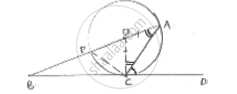Share

# In Fig.. O is the Center of the Circle and Bcd is Tangent to It at C. Prove that ∠Bac + ∠Acd = 90° - CBSE Class 10 - Mathematics

ConceptCircles Examples and Solutions

#### Question

In fig.. O is the center of the circle and BCD is tangent to it at C. Prove that ∠BAC +
∠ACD = 90°

#### Solution

Given

O is center of circle

BCD is tangent.Required to prove: ∠BAC + ∠ACD = 90°

In ΔOAC, angles opposite to equal sides are equal.

∠OAC = ∠OCA …. (i)

∠OCD = 90° [tangent is radius are perpendicular at point of contact]

∠ACD + ∠OCA = 90°

∠ACD + ∠OAC = 90° [∵ ∠OAC = ∠BAC]

∠ACD + ∠BAC = 90° ⟶ Hence proved

Is there an error in this question or solution?

#### Video TutorialsVIEW ALL 

Solution In Fig.. O is the Center of the Circle and Bcd is Tangent to It at C. Prove that ∠Bac + ∠Acd = 90° Concept: Circles Examples and Solutions.
S